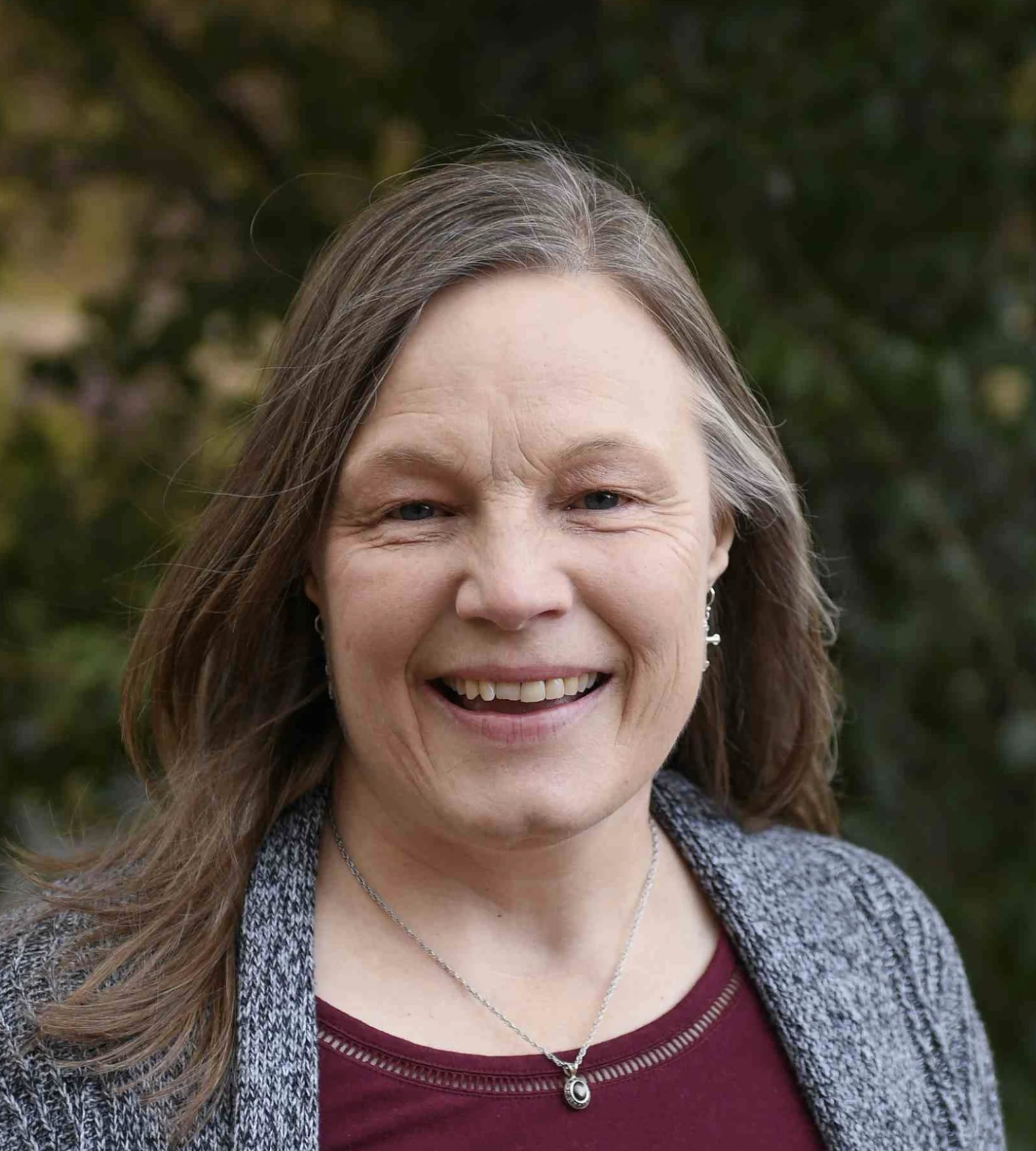# Algebra 1

\$550.00/year
Algebra 1
This class is currently archived, but if you're interested in it being taught again, you can express your interest here!
09/06/2021 - 05/14/2022
Full Year
1.0 credits in Mathematics

Taught by:Lori Miller

In the book Restoring All Things, Warren Cole Smith and John Stonestreet state, “…we glorify [God] by learning of Him and His world. To learn is an act of worship.” In the words of Galileo Galilei, “Mathematics is the language in which God has written the universe.” Learning mathematics is a powerful way to worship God.

Algebra I is the starting point for high school mathematics because it is a foundation for future courses in mathematics, science, and economics. In addition, algebraic thinking is a daily life skill. Thus, Algebra I students will continue to develop algebraic thinking instead of merely acquiring techniques to solve problems. Students will begin to move from a concrete understanding of mathematics to abstract generalizations. They will learn that mathematics is more than numbers and equations, that it encompasses logical, analytical, and creative thinking. They will develop abilities in problem-solving.

Topics covered will include the language and tools of algebra; equations, proportions, and percents; inequalities; functions; systems of equations and inequalities; exponents and polynomials; factoring polynomials; quadratic equations; data analysis and probability; and other algebraic concepts.

This 32-week course includes a weekly 1.5 hour recitation session. Students will be required to watch CTC videos and complete practice questions before class so they are prepared for in-class discussions and activities. Grades will be based on homework, class participation, unit tests, mid-term and final exams, and short oral presentations each semester.

### Course Objectives:

1. Students will recognize that mathematics is a beautiful God-given resource.
2. Students will learn to think algebraically.
3. Students will reinforce basic arithmetic skills and will learn algebraic concepts.
4. Students will apply mathematical concepts to real life situations.
5. Students will discuss the algebraic concepts being learned.

### Texts:

• CTC Math online resource. Access provided through the instructor at a cost of \$30.

## Course Files

Resource Curriculum